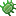# number_format

The number_format tag can be used to properly format numeric values.

For example

``````<cms:number_format '1234567890' />
``````

The snippet above will output

``````1,234,567,890.00
``````

## Parameters

• number
• decimal_precision
• decimal_character
• thousands_separator

### number

The number to be formatted.

### decimal_precision

By default two numeric characters are outputted after the decimal point. This can be changed by this parameter.

``````<cms:number_format '1234567890' decimal_precision='0' />
``````

will output

``````1,234,567,890
``````

### decimal_character

The default character used as the decimal point can be changed by this parameter.

### thousands_separator

The default character used as the thousand separator is the comma. It can be changed by this parameter.

The following snippet can be used to format a number in French notation

``````<cms:number_format '1234.56' decimal_character=',' thousands_separator=' ' />
``````

Output -

``````1 234,56
``````

## Variables

This tag is self-closing and does not set any variables of its own.